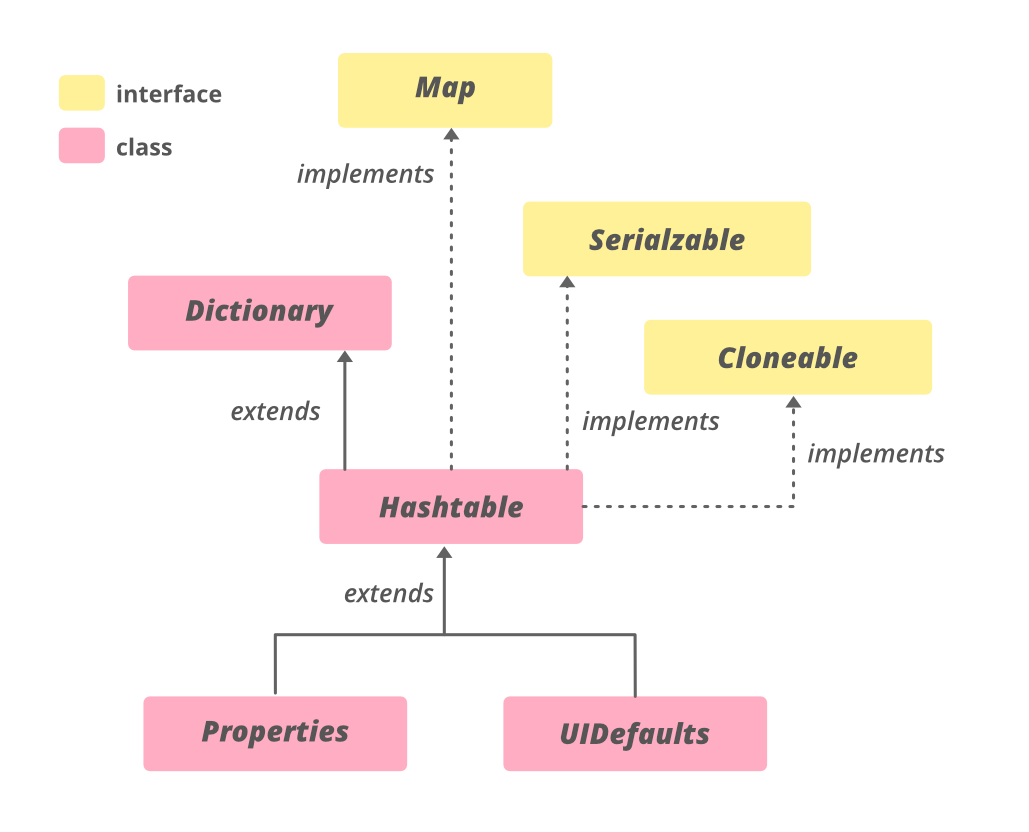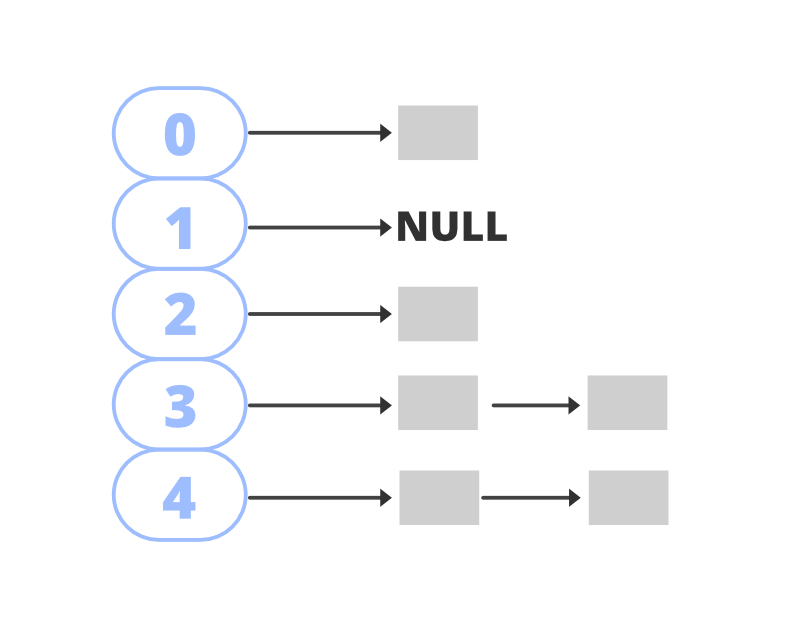Related Articles
Hashtable in Java
• Difficulty Level : Medium
• Last Updated : 24 Nov, 2020

The Hashtable class implements a hash table, which maps keys to values. Any non-null object can be used as a key or as a value. To successfully store and retrieve objects from a hashtable, the objects used as keys must implement the hashCode method and the equals method.

Features of Hashtable

• It is similar to HashMap, but is synchronized.
• Hashtable stores key/value pair in hash table.
• In Hashtable we specify an object that is used as a key, and the value we want to associate to that key. The key is then hashed, and the resulting hash code is used as the index at which the value is stored within the table.
• The initial default capacity of Hashtable class is 11 whereas loadFactor is 0.75.
• HashMap doesn’t provide any Enumeration, while Hashtable provides not fail-fast Enumeration.

Declaration:

`public class Hashtable<K,V> extends Dictionary<K,V> implements Map<K,V>, Cloneable, Serializable`

Type Parameters:

• K – the type of keys maintained by this map
• V – the type of mapped values

### The Hierarchy of HashtableHashtable implements Serializable, Cloneable, Map<K,V> interfaces and extends Dictionary<K,V>. The direct subclasses are Properties, UIDefaults.

### Constructors:

In order to create a Hashtable, we need to import it from java.util.Hashtable. There are various ways in which we can create a Hashtable.

1. Hashtable(): This creates an empty hashtable with the default load factor of 0.75 and an initial capacity is 11.

Hashtable<K, V> ht = new Hashtable<K, V>();

## Java

 `// Java program to demonstrate``// adding elements to Hashtable`` ` `import` `java.io.*;``import` `java.util.*;`` ` `class` `AddElementsToHashtable {``    ``public` `static` `void` `main(String args[])``    ``{``        ``// No need to mention the``        ``// Generic type twice``        ``Hashtable ht1 = ``new` `Hashtable<>();`` ` `        ``// Initialization of a Hashtable``        ``// using Generics``        ``Hashtable ht2``            ``= ``new` `Hashtable();`` ` `        ``// Inserting the Elements``        ``// using put() method``        ``ht1.put(``1``, ``"one"``);``        ``ht1.put(``2``, ``"two"``);``        ``ht1.put(``3``, ``"three"``);`` ` `        ``ht2.put(``4``, ``"four"``);``        ``ht2.put(``5``, ``"five"``);``        ``ht2.put(``6``, ``"six"``);`` ` `        ``// Print mappings to the console``        ``System.out.println(``"Mappings of ht1 : "` `+ ht1);``        ``System.out.println(``"Mappings of ht2 : "` `+ ht2);``    ``}``}`
Output
```Mappings of ht1 : {3=three, 2=two, 1=one}
Mappings of ht2 : {6=six, 5=five, 4=four}```

2. Hashtable(int initialCapacity): This creates a hash table that has an initial size specified by initialCapacity and the default load factor is 0.75.

Hashtable<K, V> ht = new Hashtable<K, V>(int initialCapacity);

## Java

 `// Java program to demonstrate``// adding elements to Hashtable`` ` `import` `java.io.*;``import` `java.util.*;`` ` `class` `AddElementsToHashtable {``    ``public` `static` `void` `main(String args[])``    ``{``        ``// No need to mention the``        ``// Generic type twice``        ``Hashtable ht1 = ``new` `Hashtable<>(``4``);`` ` `        ``// Initialization of a Hashtable``        ``// using Generics``        ``Hashtable ht2``            ``= ``new` `Hashtable(``2``);`` ` `        ``// Inserting the Elements``        ``// using put() method``        ``ht1.put(``1``, ``"one"``);``        ``ht1.put(``2``, ``"two"``);``        ``ht1.put(``3``, ``"three"``);`` ` `        ``ht2.put(``4``, ``"four"``);``        ``ht2.put(``5``, ``"five"``);``        ``ht2.put(``6``, ``"six"``);`` ` `        ``// Print mappings to the console``        ``System.out.println(``"Mappings of ht1 : "` `+ ht1);``        ``System.out.println(``"Mappings of ht2 : "` `+ ht2);``    ``}``}`
Output

```Mappings of ht1 : {3=three, 2=two, 1=one}
Mappings of ht2 : {4=four, 6=six, 5=five}```

3. Hashtable(int size, float fillRatio): This version creates a hash table that has an initial size specified by size and fill ratio specified by fillRatio. fill ratio: Basically, it determines how full a hash table can be before it is resized upward and its Value lies between 0.0 to 1.0.

Hashtable<K, V> ht = new Hashtable<K, V>(int size, float fillRatio);

## Java

 `// Java program to demonstrate``// adding elements to Hashtable`` ` `import` `java.io.*;``import` `java.util.*;`` ` `class` `AddElementsToHashtable {``    ``public` `static` `void` `main(String args[])``    ``{``        ``// No need to mention the``        ``// Generic type twice``        ``Hashtable ht1``            ``= ``new` `Hashtable<>(``4``, ``0``.75f);`` ` `        ``// Initialization of a Hashtable``        ``// using Generics``        ``Hashtable ht2``            ``= ``new` `Hashtable(``3``, ``0``.5f);`` ` `        ``// Inserting the Elements``        ``// using put() method``        ``ht1.put(``1``, ``"one"``);``        ``ht1.put(``2``, ``"two"``);``        ``ht1.put(``3``, ``"three"``);`` ` `        ``ht2.put(``4``, ``"four"``);``        ``ht2.put(``5``, ``"five"``);``        ``ht2.put(``6``, ``"six"``);`` ` `        ``// Print mappings to the console``        ``System.out.println(``"Mappings of ht1 : "` `+ ht1);``        ``System.out.println(``"Mappings of ht2 : "` `+ ht2);``    ``}``}`
Output
```Mappings of ht1 : {3=three, 2=two, 1=one}
Mappings of ht2 : {6=six, 5=five, 4=four}```

4. Hashtable(Map<? extends K,? extends V> m): This creates a hash table that is initialized with the elements in m.

Hashtable<K, V> ht = new Hashtable<K, V>(Map m);

## Java

 `// Java program to demonstrate``// adding elements to Hashtable`` ` `import` `java.io.*;``import` `java.util.*;`` ` `class` `AddElementsToHashtable {``    ``public` `static` `void` `main(String args[])``    ``{``        ``// No need to mention the``        ``// Generic type twice``        ``Map hm = ``new` `HashMap<>();`` ` `        ``// Inserting the Elements``        ``// using put() method``        ``hm.put(``1``, ``"one"``);``        ``hm.put(``2``, ``"two"``);``        ``hm.put(``3``, ``"three"``);`` ` `        ``// Initialization of a Hashtable``        ``// using Generics``        ``Hashtable ht2``            ``= ``new` `Hashtable(hm);`` ` `        ``// Print mappings to the console`` ` `        ``System.out.println(``"Mappings of ht2 : "` `+ ht2);``    ``}``}`
Output
`Mappings of ht2 : {3=three, 2=two, 1=one}`

Example:

## Java

 `// Java program to illustrate``// Java.util.Hashtable`` ` `import` `java.util.*;`` ` `public` `class` `GFG {``    ``public` `static` `void` `main(String[] args)``    ``{``        ``// Create an empty Hashtable``        ``Hashtable ht = ``new` `Hashtable<>();`` ` `        ``// Add elements to the hashtable``        ``ht.put(``"vishal"``, ``10``);``        ``ht.put(``"sachin"``, ``30``);``        ``ht.put(``"vaibhav"``, ``20``);`` ` `        ``// Print size and content``        ``System.out.println(``"Size of map is:- "` `+ ht.size());``        ``System.out.println(ht);`` ` `        ``// Check if a key is present and if``        ``// present, print value``        ``if` `(ht.containsKey(``"vishal"``)) {``            ``Integer a = ht.get(``"vishal"``);``            ``System.out.println(``"value for key"``                               ``+ ``" \"vishal\" is:- "` `+ a);``        ``}``    ``}``}`

Output
```Size of map is:- 3
{vaibhav=20, vishal=10, sachin=30}
value for key "vishal" is:- 10```

### Performing Various Operations on Hashtable

1. Adding Elements: In order to add an element to the hashtable, we can use the put() method. However, the insertion order is not retained in the hashtable. Internally, for every element, a separate hash is generated and the elements are indexed based on this hash to make it more efficient.

## Java

 `// Java program to demonstrate``// adding elements to Hashtable`` ` `import` `java.io.*;``import` `java.util.*;`` ` `class` `AddElementsToHashtable {``    ``public` `static` `void` `main(String args[])``    ``{``        ``// No need to mention the``        ``// Generic type twice``        ``Hashtable ht1 = ``new` `Hashtable<>();`` ` `        ``// Initialization of a Hashtable``        ``// using Generics``        ``Hashtable ht2``            ``= ``new` `Hashtable();`` ` `        ``// Inserting the Elements``          ``// using put() method``        ``ht1.put(``1``, ``"Geeks"``);``        ``ht1.put(``2``, ``"For"``);``        ``ht1.put(``3``, ``"Geeks"``);`` ` `        ``ht2.put(``1``, ``"Geeks"``);``        ``ht2.put(``2``, ``"For"``);``        ``ht2.put(``3``, ``"Geeks"``);``         ` `          ``// Print mappings to the console``        ``System.out.println(``"Mappings of ht1 : "` `+ ht1);``        ``System.out.println(``"Mappings of ht2 : "` `+ ht2);``    ``}``}`

Output
```Mappings of ht1 : {3=Geeks, 2=For, 1=Geeks}
Mappings of ht2 : {3=Geeks, 2=For, 1=Geeks}```

2. Changing Elements: After adding the elements if we wish to change the element, it can be done by again adding the element with the put() method. Since the elements in the hashtable are indexed using the keys, the value of the key can be changed by simply inserting the updated value for the key for which we wish to change.

## Java

 `// Java program to demonstrate``// updating Hashtable`` ` `import` `java.io.*;``import` `java.util.*;``class` `UpdatesOnHashtable {``    ``public` `static` `void` `main(String args[])``    ``{`` ` `        ``// Initialization of a Hashtable``        ``Hashtable ht``            ``= ``new` `Hashtable();`` ` `        ``// Inserting the Elements``          ``// using put method``        ``ht.put(``1``, ``"Geeks"``);``        ``ht.put(``2``, ``"Geeks"``);``        ``ht.put(``3``, ``"Geeks"``);``         ` `          ``// print initial map to the console``        ``System.out.println(``"Initial Map "` `+ ht);``         ` `          ``// Update the value at key 2``        ``ht.put(``2``, ``"For"``);``         ` `          ``// print the updated map``        ``System.out.println(``"Updated Map "` `+ ht);``    ``}``}`

Output
```Initial Map {3=Geeks, 2=Geeks, 1=Geeks}
Updated Map {3=Geeks, 2=For, 1=Geeks}```

3. Removing Element: In order to remove an element from the Map, we can use the remove() method. This method takes the key value and removes the mapping for a key from this map if it is present in the map.

## Java

 `// Java program to demonstrate``// the removing mappings from Hashtable`` ` `import` `java.io.*;``import` `java.util.*;``class` `RemovingMappingsFromHashtable {`` ` `    ``public` `static` `void` `main(String args[])``    ``{``        ``// Initialization of a Hashtable``        ``Map ht``            ``= ``new` `Hashtable();`` ` `        ``// Inserting the Elements``          ``// using put method``        ``ht.put(``1``, ``"Geeks"``);``        ``ht.put(``2``, ``"For"``);``        ``ht.put(``3``, ``"Geeks"``);``        ``ht.put(``4``, ``"For"``);`` ` `        ``// Initial HashMap``        ``System.out.println(``"Initial map : "` `+ ht);`` ` `          ``// Remove the map entry with key 4``        ``ht.remove(``4``);`` ` `        ``// Final Hashtable``        ``System.out.println(``"Updated map : "` `+ ht);``    ``}``}`
Output
```Initial map : {4=For, 3=Geeks, 2=For, 1=Geeks}
Updated map : {3=Geeks, 2=For, 1=Geeks}```

4. Traversal of a Hashtable: To iterate the table, we can make use of an advanced for loop. Below is the example of iterating a hashtable.

## Java

 `// Java program to illustrate``// traversal of Hashtable`` ` `import` `java.util.Hashtable;``import` `java.util.Map;`` ` `public` `class` `IteratingHashtable {``    ``public` `static` `void` `main(String[] args)``    ``{``          ``// Create an instance of Hashtable``        ``Hashtable ht = ``new` `Hashtable<>();`` ` `          ``// Adding elements using put method``        ``ht.put(``"vishal"``, ``10``);``        ``ht.put(``"sachin"``, ``30``);``        ``ht.put(``"vaibhav"``, ``20``);``     ` `          ``// Iterating using enhanced for loop``        ``for` `(Map.Entry e : ht.entrySet())``            ``System.out.println(e.getKey() + ``" "``                               ``+ e.getValue());``    ``}``}`
Output
```vaibhav 20
vishal 10
sachin 30```

### Internal Working of Hashtable

Hashtable datastructure is an array of buckets which stores the key/value pairs in them. It makes use of hashCode() method to determine which bucket the key/value pair should map.
The hash function helps to determine the location for a given key in the bucket list. Generally, hashcode is a non-negative integer that is equal for equal Objects and may or may not be equal for unequal Objects. To determine whether two objects are equal or not, hashtable makes use of the equals() method.

It is possible that two unequal Objects have the same hashcode. This is called a collision. To resolve collisions, hashtable uses an array of lists. The pairs mapped to a single bucket (array index) are stored in a list and list reference is stored in the array index.### Methods of Hashtable

• K – The type of the keys in the map.
• V – The type of values mapped in the map.